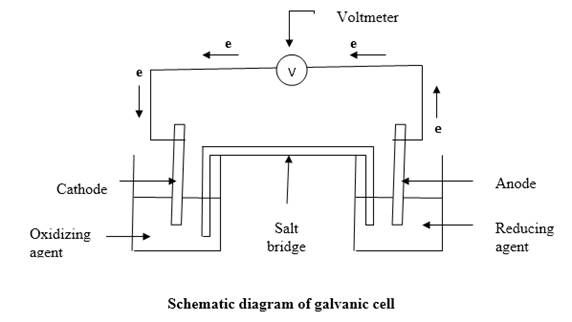# Sketch a galvanic cell, and explain how it works. Look at Figs. 18.1 and 18.5. Explain what is occurring in each container and why the cell in Fig. 18.5 “works,” but the one in Fig. 18.1 does not.### Introductory Chemistry: A Foundati...

9th Edition
Steven S. Zumdahl + 1 other
Publisher: Cengage Learning
ISBN: 9781337399425### Introductory Chemistry: A Foundati...

9th Edition
Steven S. Zumdahl + 1 other
Publisher: Cengage Learning
ISBN: 9781337399425

#### Solutions

Chapter 18, Problem 1ALQ
Textbook Problem

## Sketch a galvanic cell, and explain how it works. Look at Figs. 18.1 and 18.5. Explain what is occurring in each container and why the cell in Fig. 18.5 “works,” but the one in Fig. 18.1 does not.

Expert Solution
Interpretation Introduction

Interpretation: A galvanic cell should be sketched and how it works should be explained. Considering the figures 18.1 and 18.5 , what is occurring in each container should be explained and why the cell in figure 18.5 works, but the one in figure 18.1 does not should be discussed.

Concept Introduction:A Galvanic cell also known as electrochemical battery, a device that exploits a spontaneous oxidation reduction reaction to produce electrical energy. Here oxidizing agent and reducing agent has been separated, so that the electron/s generated by oxidation should travel through a wire from reducing agent to oxidizing agent.

### Explanation of Solution

The galvanic cell is sketched as follows:When reducing agent and oxidizing agent mixed together in a container, electron transfer occurs. But chemical energy generated by this cannot be used for any work. This can be achieved by separating the reducing agent and oxidizing agent and let the electron transfer happen through a wire. So electrons removing from the reducing agent will travel through the wire to the oxidizing agent. The electric current generated by the electron flow through the wire can be directed through an electronic device. To keep the net charge of each container zero, the two solutions are connected by a salt bridge which filled with strong electrolyte.

In the figure 18.1 , the beaker in the left contains the oxidizing agent, MnO42-, which undergoes reduction by accepting electrons and forms Mn2+. The beaker in the right contains the reducing agent, Fe2+ which undergoes oxidation by removing electrons and converts into Fe3+.

According to the figure 18.1 , electrons flow only from reducing agent to the oxidizing agent. this will build up a negative charge in the left container and a positive charge in the right container. This will create a charge separation and to create charge separation large amount of energy is needed. So, no electron flow occurs under these conditions. This problem has been solved in the figure 18.5 . The solutions have been connected through a salt bridge without mixing the two solutions. Therefore the electrons from the reducing agent will flow through the wire to the oxidizing agent and the ions in the aqueous solution flows from one container to the other through salt bridge keeping the net charge of the each container zero.

### Want to see more full solutions like this?

Subscribe now to access step-by-step solutions to millions of textbook problems written by subject matter experts!

### Want to see more full solutions like this?

Subscribe now to access step-by-step solutions to millions of textbook problems written by subject matter experts!